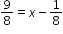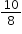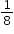# MA.6.AR.2.4Export Print
Determine the unknown decimal or fraction in an equation involving any of the four operations, relating three numbers, with the unknown in any position.

### Examples

Given the equation, x can be determined to bebecauseismore than.

### Clarifications

Clarification 1: Instruction focuses on using algebraic reasoning, drawings, and mental math to determine unknowns.

Clarification 2: Problems include the unknown and different operations on either side of the equal sign. All terms and solutions are limited to positive rational numbers.

General Information
Subject Area: Mathematics (B.E.S.T.)
Strand: Algebraic Reasoning
Status: State Board Approved

## Related Courses

This benchmark is part of these courses.
1205010: M/J Grade 6 Mathematics (Specifically in versions: 2014 - 2015, 2015 - 2022, 2022 and beyond (current))
1205020: M/J Accelerated Mathematics Grade 6 (Specifically in versions: 2014 - 2015, 2015 - 2020, 2020 - 2022, 2022 and beyond (current))
1204000: M/J Foundational Skills in Mathematics 6-8 (Specifically in versions: 2014 - 2015, 2015 - 2022, 2022 and beyond (current))
7812015: Access M/J Grade 6 Mathematics (Specifically in versions: 2014 - 2015, 2015 - 2018, 2018 - 2022, 2022 and beyond (current))

## Related Access Points

Alternate version of this benchmark for students with significant cognitive disabilities.
MA.6.AR.2.AP.4: Solve a one-step equation using fractions with like denominators or decimals with place value ranging from the thousand to the thousandths.

## Related Resources

Vetted resources educators can use to teach the concepts and skills in this benchmark.

## Formative Assessment

Solar Solutions:

Students are asked to solve a real-world problem by writing and solving an equation.

Type: Formative Assessment

## Tutorial

How to Solve One-Step Multiplication and Division Equations with Fractions and Decimals:

In this tutorial, we will solve equations in one step by multiplying or dividing a number on both sides.

Type: Tutorial

## MFAS Formative Assessments

Solar Solutions:

Students are asked to solve a real-world problem by writing and solving an equation.

## Student Resources

Vetted resources students can use to learn the concepts and skills in this benchmark.

## Tutorial

How to Solve One-Step Multiplication and Division Equations with Fractions and Decimals:

In this tutorial, we will solve equations in one step by multiplying or dividing a number on both sides.

Type: Tutorial

## Parent Resources

Vetted resources caregivers can use to help students learn the concepts and skills in this benchmark.